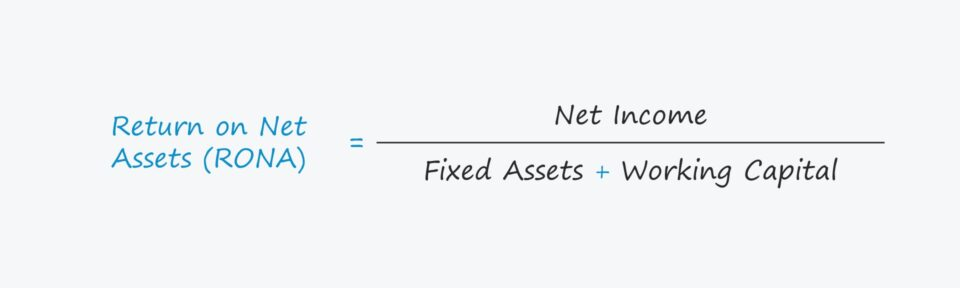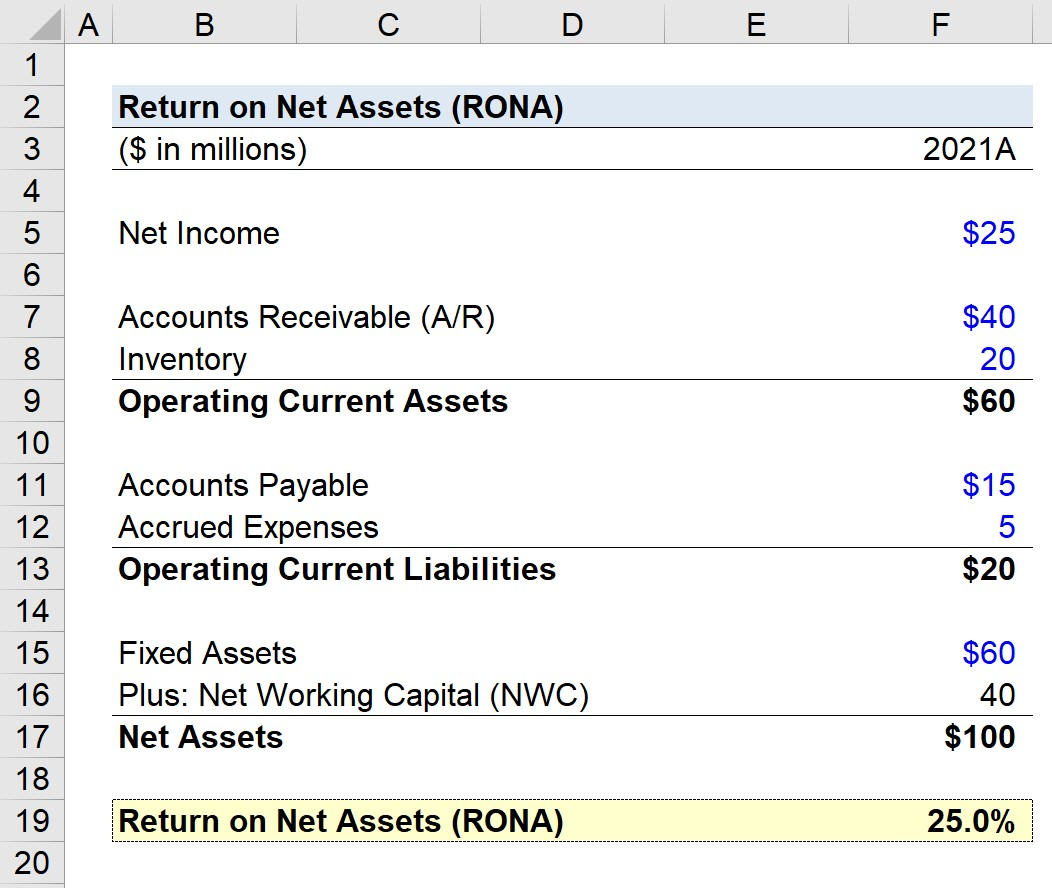Welcome to Wall Street Prep! Use code at checkout for 15% off.# Return on Net Assets (RONA)

Guide to Understanding Return on Net Assets (RONA)## How to Calculate Return on Net Assets (Step-by-Step)

RONA stands for “return on net assets” and is used to determine if management is allocating its net assets effectively to generate more earnings.

There are three inputs necessary to calculate the return on net assets (RONA):

By dividing net income by the sum of fixed assets and net working capital (NWC), the return on net assets (RONA) metric answers: “How much is the company earning in net profits per dollar of fixed assets and net assets owned?”

With that said, the higher the RONA, the more efficient the company is at generating profits (and vice versa).

The “net assets” metric is comprised of two items:

1. Fixed Assets → The long-term tangible assets belonging to a company that is expected to provide economic benefits in excess of one year, i.e. property, plant and equipment (PP&E).
2. Net Working Capital (NWC) → The difference between operating current assets and operating current liabilities.

While the fixed assets (PP&E) component is relatively intuitive, the net working capital (NWC) metric is a variation of the traditional working capital formula taught in academia.

In this calculation, net working capital (NWC) includes only the operating current assets and operating current liabilities.

The notable adjustment here is that cash and cash equivalents, as well as debt and any interest-bearing securities, are removed and are not part of the net working capital (NWC) calculation.

Neither cash nor debt represents operating items that directly contribute to future revenue generation, hence their removal from the operating working capital (OWC) metric.

## Return on Net Assets Formula

The formula for calculating the return on net assets (RONA) is as follows.

Return on Net Assets (RONA) = Net Income ÷ (Fixed Assets + Net Working Capital)

Net income, i.e. the “bottom line”, is found on the income statement.

On the other hand, the carrying values of a company’s fixed assets (PP&E) and net working capital (NWC) can be found on the balance sheet.

Ensure that the net working capital (NWC) consists only of operating current assets, subtracted by operating current liabilities.

###### Average vs. Ending Balance Sheet Values

In order to match the numerator and denominator in terms of timing (i.e. for the income statement vs. balance sheet), the average balance could technically be used for the fixed assets and net working capital (NWC) calculation.

However, barring unusual circumstances, it is still acceptable to use the ending balance in most cases, as the difference between calculations is usually negligible.

## Return on Net Assets (RONA) vs. Return on Assets (ROA)

The return on assets (ROA) measures the efficiency at which a company utilizes its asset base to generate net profits.

Like the return on net assets (RONA) metric, the return on assets (ROA) is used for tracking how efficiently a company puts its assets to use – albeit, ROA is far more common to see in practice.

For either metric, the higher the return, the more efficient the company is operating since its assets are utilized near full capacity (and are closer to reaching their “ceiling” for attainable net profits).

The formula used to calculate the return on assets (ROA) can be found below.

Return on Assets (ROA) = Net Income ÷ Average Total Assets

The numerator is also net income, but the distinction is the denominator, which consists of the average value of a company’s entire asset base.

The RONA metric is therefore a variation of ROA, where non-operating assets are intentionally excluded.

In a sense, RONA normalizes the assets taken into account and makes comparisons among different companies more informative (and closer to being “apples to apples”).

Since the end goal is to determine how well management is utilizing its assets, it is arguably more logical to isolate fixed assets (PP&E) and net assets.

## Return on Net Assets Calculator – Excel Model Template

We’ll now move to a modeling exercise, which you can access by filling out the form below.Submitting...

## Return on Net Assets Calculation Example

Suppose a company generated \$25 million in net income for the fiscal year ending 2021.

For our net working capital (NWC) schedule, we’ll assume the following carrying values:

• Accounts Receivable (A/R) = \$40 million
• Inventory = \$20 million
• Operating Current Assets = \$60 million
• Accounts Payable = \$15 million
• Accrued Expenses = \$5 million
• Operating Current Liabilities = \$20 million

Using those figures, our company’s net working capital (NWC) comes out to \$40 million, which we calculated by subtracting the operating current liabilities (\$20 million) from the operating current assets (\$60 million).

• Net Working Capital (NWC) = \$60 million – \$40 million = \$20 million

Here, we’re using the ending balances rather than the average balance for the sake of simplicity.

The only input remaining is the fixed assets balance, which we’ll assume to be \$60 million.

• Fixed Assets = \$60 million

Therefore, the company’s net assets are worth \$100 million, while its net income is \$25 million.

In closing, upon dividing our company’s net income (\$25 million) by the value of its net assets (\$100 million), we arrive at an implied return on net assets (RONA) of 25%.

• Return on Net Assets (RONA) = \$25 million ÷ (\$60 million + \$40 million) = 0.25, or 25%Step-by-Step Online Course

### Everything You Need To Master Financial Modeling

Enroll in The Premium Package: Learn Financial Statement Modeling, DCF, M&A, LBO and Comps. The same training program used at top investment banks.